# Difference between revisions of "Permanent"

of an $m \times n$-matrix $A = \left\Vert a_{ij} \right\Vert$

The function $$\mathrm{per}(A) = \sum_\sigma a_{1\sigma(1)}\cdots a_{m\sigma(m)}$$

where $a_{ij}$ are elements from a commutative ring and summation is over all one-to-one mappings $\sigma$ from $\{1,\ldots,m\}$ into $\{1,\ldots,n\}$. If $m=n$, then $\sigma$ represents all possible permutations, and the permanent is a particular case of the Schur matrix function (cf. Immanant) $$d_\chi^H (A) = \sum_{\sigma\in H} \chi(\sigma) \prod_{i=1}^n a_{i\sigma(i)}$$ for $H \subseteq S_n$, where $\chi$ is a character of degree 1 on the subgroup $H$ (cf. Character of a group) of the symmetric group $S_n$ (one obtains the determinant for $H=S_n$, $\chi =\pm 1$, in accordance with the parity of $\sigma$).

The permanent is used in linear algebra, probability theory and combinatorics. In combinatorics, a permanent can be interpreted as follows: The number of systems of distinct repesentatives for a given family of subsets of a finite set is the permanent of the incidence matrix for the incidence system related to this family.

The main interest is in the permanent of a matrix consisting of zeros and ones (a $(0,1)$-matrix), of a matrix containing non-negative real numbers, in particular doubly-stochastic matrices (in which the sum of the elements in any row and any column is 1), and of a complex Hermitian matrix. The basic properties of the permanent include a theorem on expansion (the analogue of Laplace's theorem for determinants) and the Binet–Cauchy theorem, which gives a representation of the permanent of the product of two matrices as the sum of the products of the permanents formed from the cofactors. For the permanents of complex matrices it is convenient to use representations as scalar products in the symmetry classes of completely-symmetric tensors (see, e.g., ). One of the most effective methods for calculating permanents is provided by Ryser's formula: $$\mathrm{per}(A) = \sum_{t=0}^{n-1} (-1)^t \sum_{X \in \Gamma_{n-t}} \prod_{i=1}^m r_i(X)$$ where $\Gamma_k$ is the set of submatrices of dimension $m \times k$ for the square matrix $A$, $r_i = r_i(X)$ is the sum of the elements of the $i$-th row of $X$ and $i,k=1,\ldots,m$.

As it is complicated to calculate permanents, estimating them is important. Some lower bounds are given below.

a) Ifis a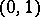-matrix with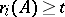,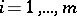, then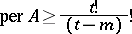for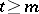, and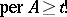ifand.

b) Ifis a-matrix of order, thenwhere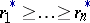are the sums of the elements in the rows ofarranged in non-increasing order and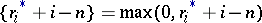.

c) Ifis a positive semi-definite Hermitian matrix of order, thenwhere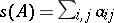if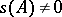.

Upper bounds for permanents:

1) For a-matrixof order,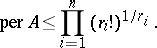2) For a completely-indecomposable matrixof orderwith non-negative integer elements,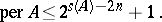3) For a complex normal matrixwith eigen values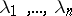,The most familiar problem in the theory of permanents was van der Waerden's conjecture: The permanent of a doubly-stochastic matrix of orderis bounded from below by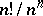, and this value is attained only for the matrix composed of fractions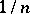. A positive solution to this problem was obtained in .

Among the applications of permanents one may mention relationships to certain combinatorial problems (cf. Combinatorial analysis), such as the "problème des rencontresproblème de rencontres" and the "problème d'attachementproblème d'attachement" (or "hook problemhook problem" ), and also to the Fibonacci numbers, the enumeration of Latin squares (cf. Latin square) and Steiner triple systems (cf. Steiner system), and to the derivation of the number of-factors and linear subgraphs of a graph, while doubly-stochastic matrices are related to certain probability models. There are interesting physical applications of permanents, of which the most important is the dimer problem, which arises in research on the adsorption of di-atomic molecules in surface layers: The permanent of a-matrix of a simple structure expresses the number of ways of combining the atoms in the substance into di-atomic molecules. There are also applications of permanents in statistical physics, the theory of crystals and physical chemistry.